Area Of Irregular Shapes - Definition with Examples

The Complete K-5 Math Learning Program Built for Your Child

• 30 Million Kids

Loved by kids and parent worldwide

• 50,000 Schools

Trusted by teachers across schools

• Comprehensive Curriculum

Aligned to Common Core

Area of Irregular Shapes

Irregular shapes are the polygons with five or more sides of varying lengths. These shapes or figures can be decomposed further into triangles, squares, and quadrilaterals to evaluate the area.

Some examples of irregular shapes are as follows: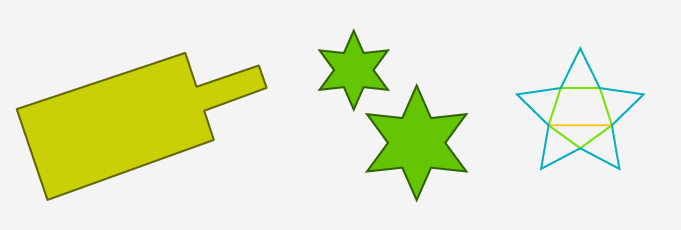Daily life objects with irregular shapes

Calculating the area of irregular shapes:

The approaches to estimating the area of irregular shape are:

Evaluating area using unit squares

Apply this technique for the shapes with curves apart from perfect circle or semicircles and irregular quadrilaterals. In this method, divide the shape into unit squares. The total number of unit squares falling within the shape determines the total area.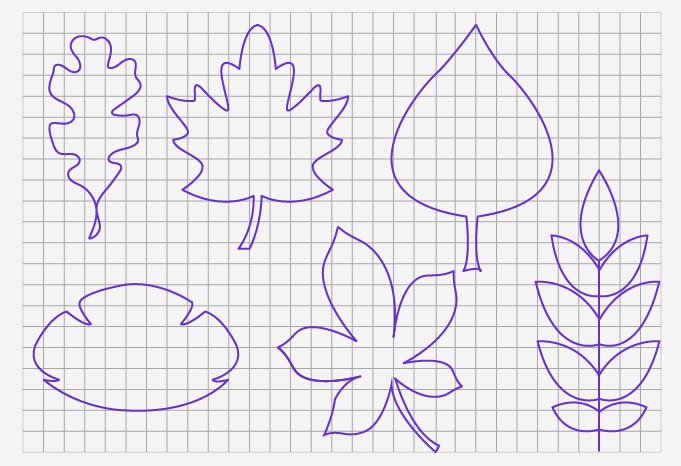Figure: Some examples of irregular shapes

Count the square as “1” if the shaded region covers more than half while calculating the area for a more accurate estimate.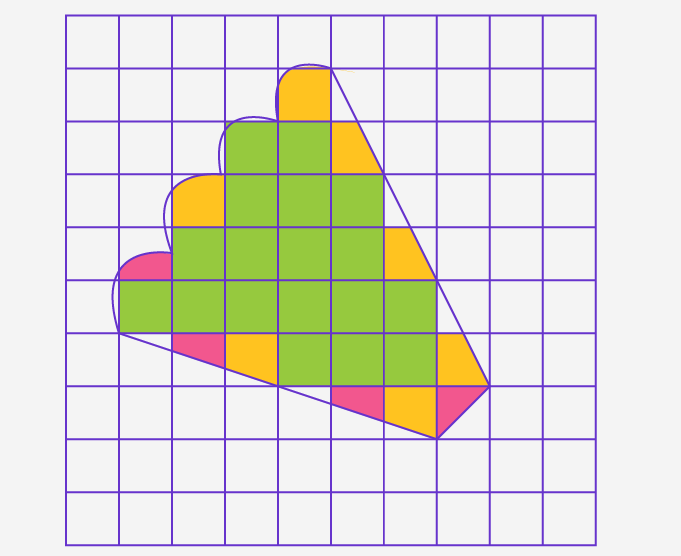Figure: For the irregular shape, count the squares with orange and yellow coding as 1.

In the following figure, calculate the area by counting the unit squares, which is 6. If we denote each unit square in centimeter, the area will be 6 cm2.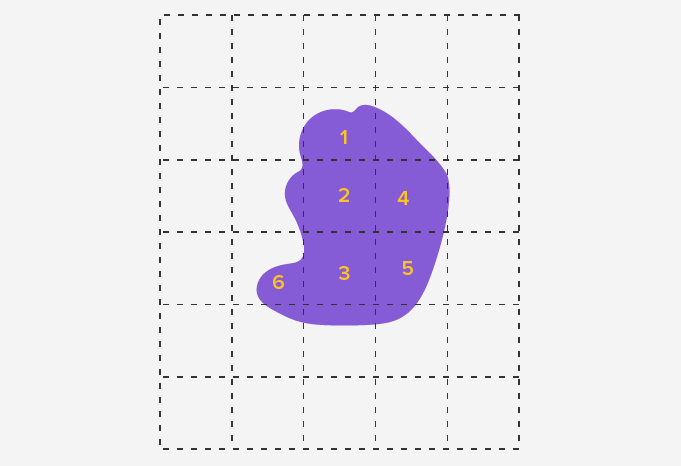Figure: Calculating the area of an irregular shape with curved edges

• Dividing the irregular shape in two or more regular shapes

Use this method for irregular shapes, which are a combination of triangles and polygons. Use predefined formulas to calculate the area of such shapes and add them together to obtain the total area.

For example, an irregular shape we divide multiple edges into a triangle and three polygons.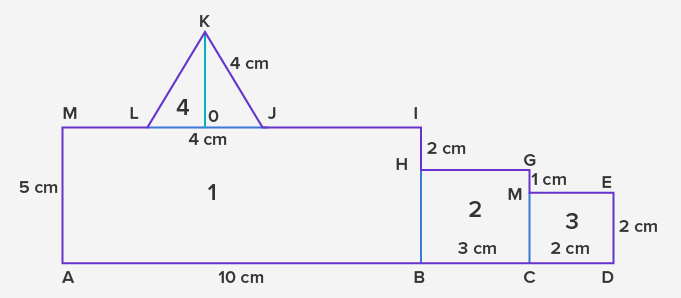The total area of the figure is given as:

⇒ Area = Area (ABIM) + Area (BCGH) + Area (CDEF) + Area (JKL)

⇒ Area = (AB × BI) + (BC × CG) + (CD × DE) + (12× LJ × KO)

⇒ Area = ( 10 × 5) + (3 × 3) + (2 × 2) + (12× 4 × 4)

⇒ Area = 50 + 9 + 4 + 8

⇒ Area = 71 cm2

• Dividing the irregular shape with curves in two or more regular shapes

In this method, decompose an irregular shape into multiple squares, triangles, or other quadrilaterals. Depending on the shape and curves, a part of the figure can be a circle, semicircle or quadrant as well.

The following figure is an irregular shape with 8 sides, including one curve. Determine the unknown quantities by the given dimensions for the sides. Decompose the figure into two rectangles and a semicircle.

The area of the shape ABCDEF is:

Area (ABCDEF) = Area (ABCG) + Area (GDEF) + Area (aob)

Area = (AB × AG) + (GD × DE) + (12 × π × ob2)

Area = (3 × 4) + (10 × 4) + (12 × 3.14 × 12)

Area = 12 + 40 + 1.57

Area = 53.57 cm2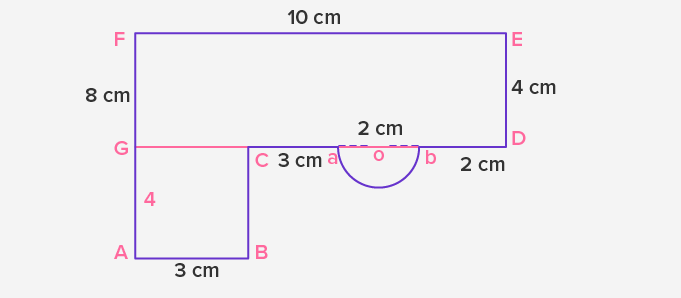Application

The estimation of area for irregular figures is an essential method for drawing maps, building architecture, and marking agricultural fields. We apply the concept in the cutting of fabrics as per the given design. In higher grades, the technique lays a basis for advanced topics such as calculating volume, drawing conic sections and figures with elliptical shapes.

 Fun Fact Tangram polygons are ancient examples of geometric shapes and can create more than 6500 shapes.Related math vocabulary

• Square
• Rectangle
• Triangle
• Circle
• Area
• Irregular and regular shapes
Won Numerous Awards & Honors